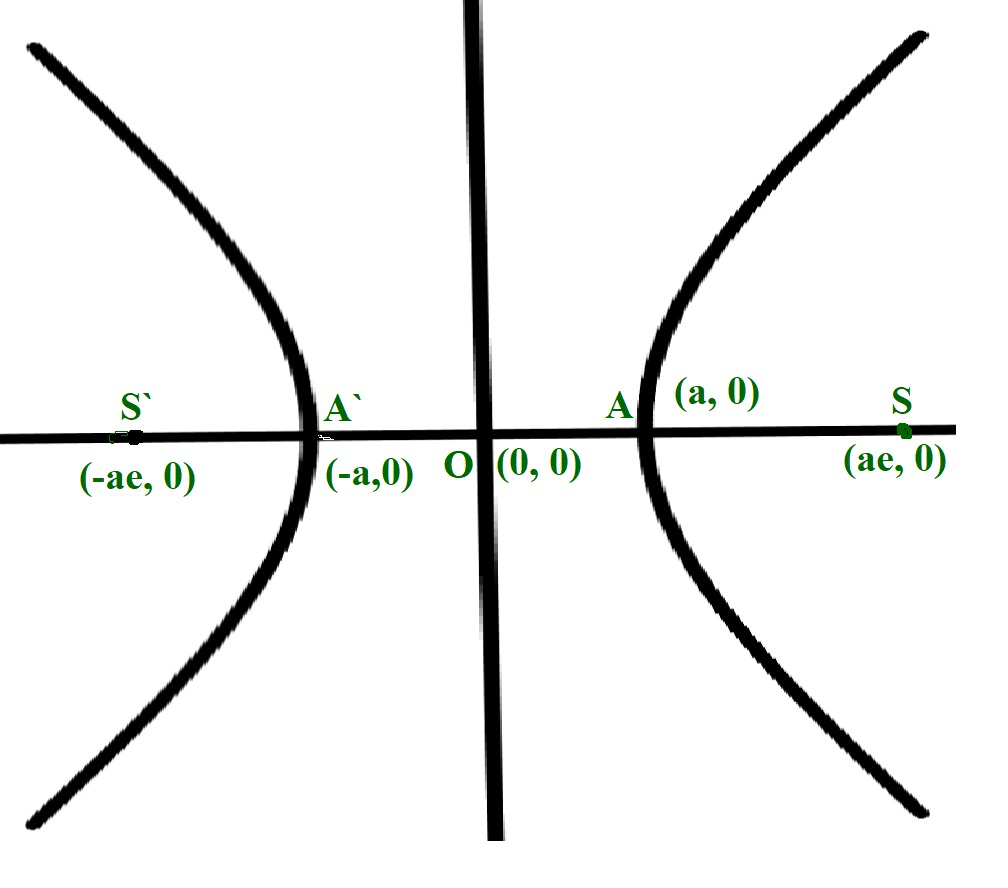Open in App
Not now

# Program to find the Eccentricity of a Hyperbola

• Last Updated : 13 May, 2021

Given two integers A and B, representing the length of the semi-major and semi-minor axis of a Hyperbola of the equation (X2 / A2) – (Y2 / B2) = 1, the task is to calculate the eccentricity of the given hyperbola.

Examples:

Input: A = 3, B = 2
Output: 1.20185
Explanation:
The eccentricity of the given hyperbola is 1.20185.

Input: A = 6, B = 3
Output: 1.11803

Approach: The given problem can be solved by using the formula to find the eccentricity of an ellipse.• The length of the semi-major axis is A.
• The length of the semi-minor axis is B.
• Therefore, the eccentricity of the ellipse is given bywhere A > B

Therefore, the idea is to print the value ofas the eccentricity of the ellipse.

Below is the implementation of the above approach:

## C++

 // C++ program for the above approach   #include  using namespace std;   // Function to find the eccentricity // of a hyperbola double eccHyperbola(double A, double B) {     // Stores the squared ratio     // of major axis to minor axis     double r = (double)B * B / A * A;       // Increment r by 1     r += 1;       // Return the square root of r     return sqrt(r); }   // Driver Code int main() {     double A = 3.0, B = 2.0;     cout << eccHyperbola(A, B);       return 0; }

## Java

 // Java program for the above approach import java.util.*;   class GFG{   // Function to find the eccentricity // of a hyperbola static double eccHyperbola(double A, double B) {           // Stores the squared ratio     // of major axis to minor axis     double r = (double)B * B / A * A;       // Increment r by 1     r += 1;       // Return the square root of r     return Math.sqrt(r); }   // Driver Code public static void main(String[] args) {     double A = 3.0, B = 2.0;           System.out.print(eccHyperbola(A, B)); } }   // This code is contributed by Amit Katiyar

## Python3

 # Python3 program for the above approach import math   # Function to find the eccentricity # of a hyperbola     def eccHyperbola(A, B):       # Stores the squared ratio     # of major axis to minor axis     r = B * B / A * A       # Increment r by 1     r += 1       # Return the square root of r     return math.sqrt(r)     # Driver Code if __name__ == "__main__":       A = 3.0     B = 2.0     print(eccHyperbola(A, B))       # This code is contributed by ukasp

## C#

 // C# program for the above approach using System;   class GFG{   // Function to find the eccentricity // of a hyperbola static double eccHyperbola(double A, double B) {           // Stores the squared ratio     // of major axis to minor axis     double r = (double)B * B / A * A;       // Increment r by 1     r += 1;       // Return the square root of r     return Math.Sqrt(r); }   // Driver Code public static void Main(String[] args) {     double A = 3.0, B = 2.0;           Console.Write(eccHyperbola(A, B)); } }   // This code is contributed by Princi Singh

## Javascript

 

Output:

2.23607

Time Complexity: O(1)
Auxiliary Space: O(1)

My Personal Notes arrow_drop_up
Related Articles